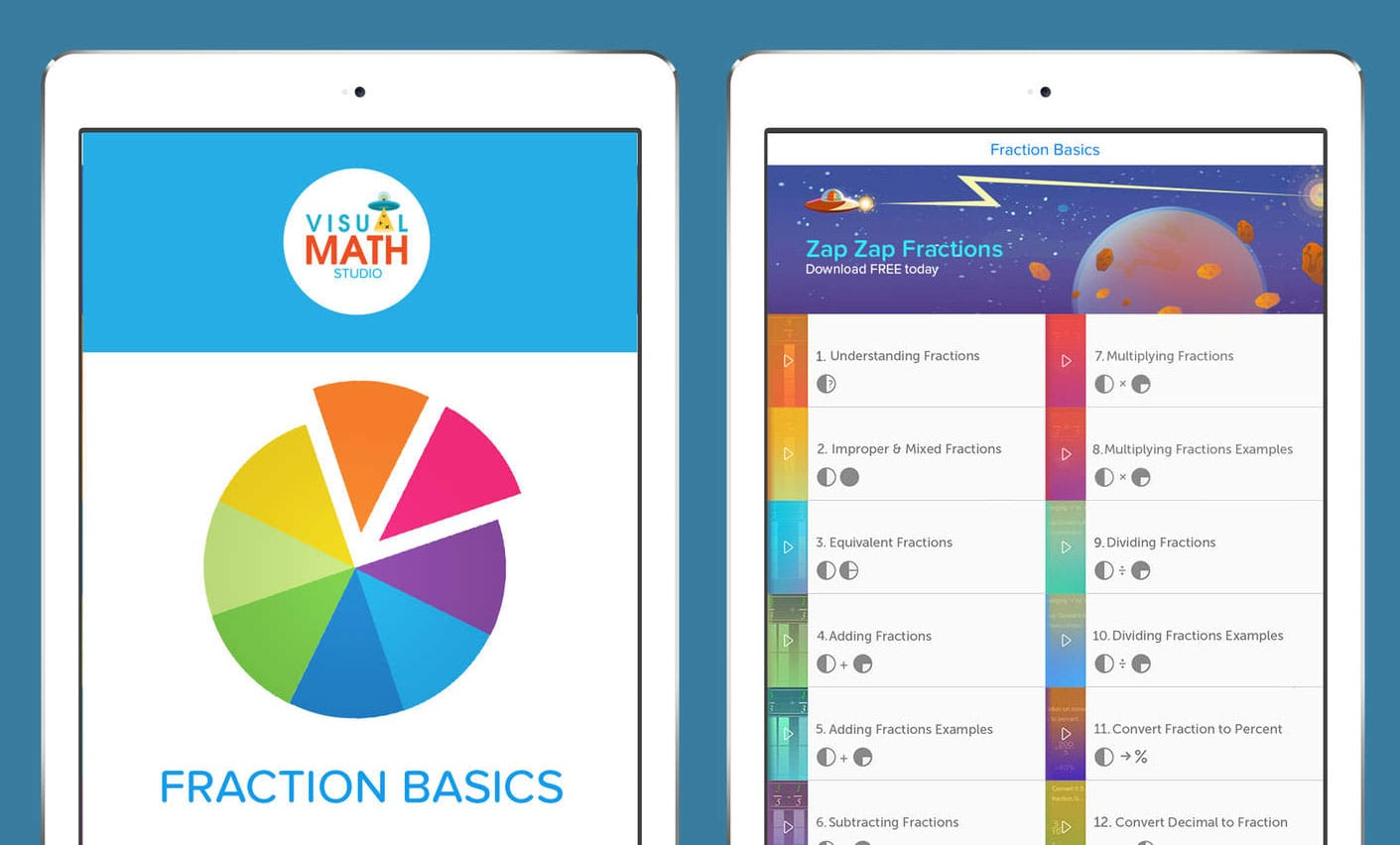# Fraction Basics: Adding and subtracting fractions explained with videoFraction Basics covers everything you need to know about adding and subtracting fractions, as well as multiplying and dividing. In addition to that, it also explains how to convert fractions to percentages and decimals to fractions.

What’s great about Fraction Basics is that you learn through interactive videos and easy-to-follow steps. There are 12 videos in total and you can skip through steps if need be.

The topics covered are:

1. Understanding Fractions
2. Improper and Mixed Fractions
3. Equivalent Fractions
6. Subtracting Fractions
7. Multiplying Fractions
8. Multiplying Fractions Examples
9. Dividing Fractions
10. Dividing Fractions Examples
11. Convert Fraction to Percent
12. Convert Decimal to Fraction

The app is free and is available to download for iOS devices.

SaveSave

SaveSave

Cool
0
Excited
0
Happy
0
Love
0
Wow
0